1G3.1
 Name:    1G3.1

Multiple Choice
Identify the choice that best completes the statement or answers the question.

1.

Which of the following shapes shows 2 quarters shaded?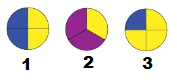a. 1 c. 3 b. 2

2.

Which of the following shapes shows 2 quarters shaded?a. 1 c. 3 b. 2

3.

How much of a dollar is \$.25
 a. a fifth c. a half b. a quarter

4.

This is a dollar.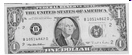How many quarters are there in a dollar?
 a.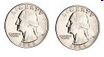c.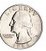b.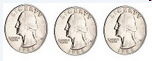d.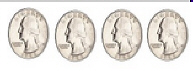5.

Which one of these shapes has a fourth of it shaded?
 a.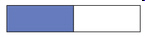c.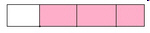b.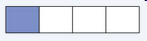6.

Which of these pictures has half of its area shaded?
 a.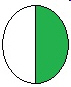c.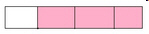b.7.

What word describes how this circle is divided?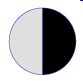a. quarters c. halves b. fourths

8.

Which circle is divided into quarters?
 a.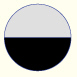c.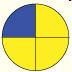b.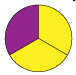9.

Which rectangle below is divided into halves?
 a.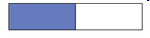c.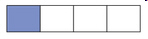b.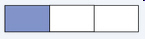10.

What does the shaded area of this rectangle show?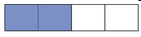a. quarter of c. fourth of b. half of